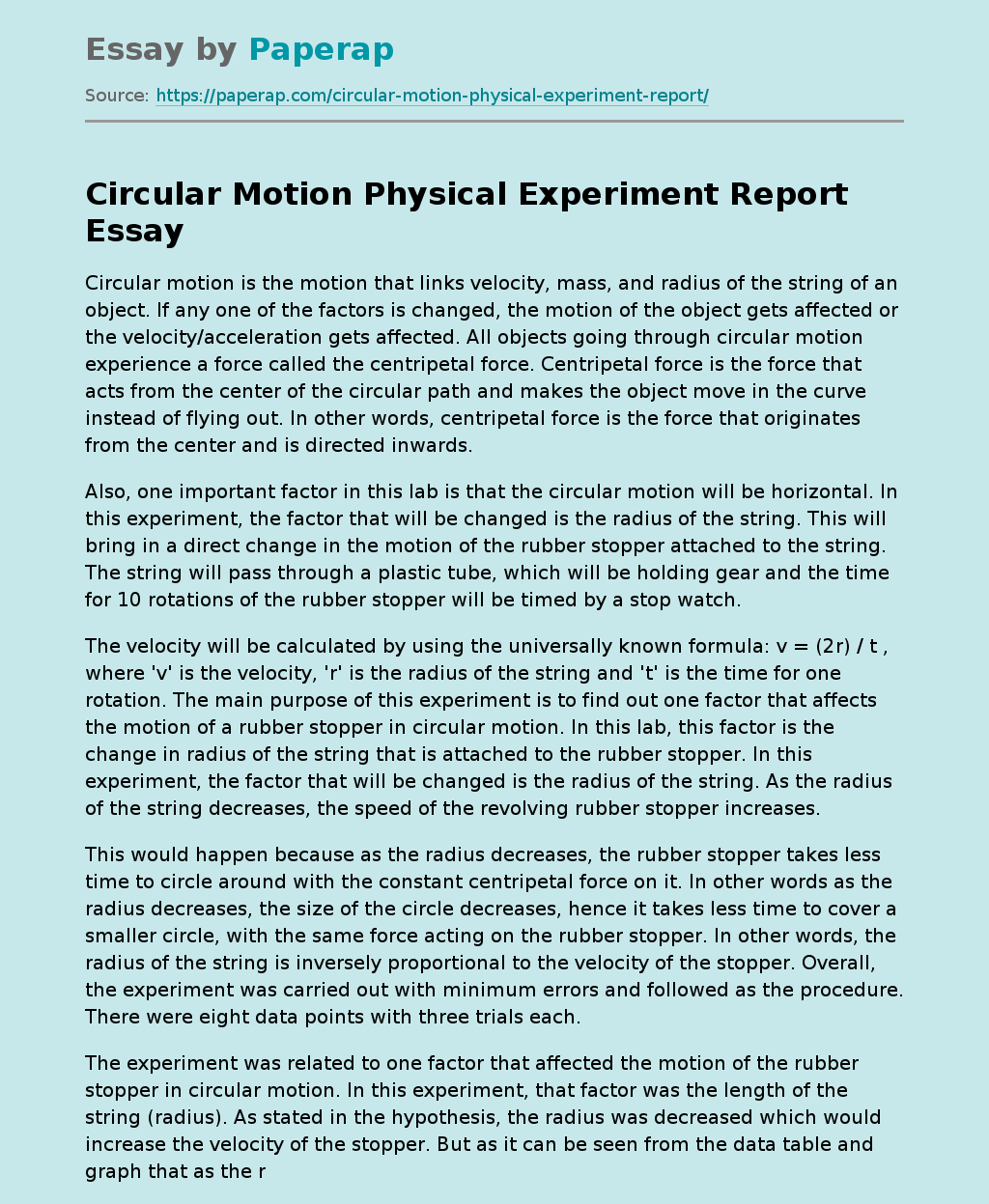# Circular Motion Physical Experiment Report

Circular motion is the motion that links velocity, mass, and radius of the string of an object. If any one of the factors is changed, the motion of the object gets affected or the velocity/acceleration gets affected. All objects going through circular motion experience a force called the centripetal force. Centripetal force is the force that acts from the center of the circular path and makes the object move in the curve instead of flying out. In other words, centripetal force is the force that originates from the center and is directed inwards.

Also, one important factor in this lab is that the circular motion will be horizontal. In this experiment, the factor that will be changed is the radius of the string.

Get quality help nowRhizManVerified

Proficient in: Force4.9 (247)

“ Rhizman is absolutely amazing at what he does . I highly recommend him if you need an assignment done ”+84 relevant experts are online

This will bring in a direct change in the motion of the rubber stopper attached to the string. The string will pass through a plastic tube, which will be holding gear and the time for 10 rotations of the rubber stopper will be timed by a stop watch.

The velocity will be calculated by using the universally known formula: v = (2r) / t , where ‘v’ is the velocity, ‘r’ is the radius of the string and ‘t’ is the time for one rotation. The main purpose of this experiment is to find out one factor that affects the motion of a rubber stopper in circular motion. In this lab, this factor is the change in radius of the string that is attached to the rubber stopper. In this experiment, the factor that will be changed is the radius of the string. As the radius of the string decreases, the speed of the revolving rubber stopper increases.

This would happen because as the radius decreases, the rubber stopper takes less time to circle around with the constant centripetal force on it. In other words as the radius decreases, the size of the circle decreases, hence it takes less time to cover a smaller circle, with the same force acting on the rubber stopper. In other words, the radius of the string is inversely proportional to the velocity of the stopper. Overall, the experiment was carried out with minimum errors and followed as the procedure. There were eight data points with three trials each.

The experiment was related to one factor that affected the motion of the rubber stopper in circular motion. In this experiment, that factor was the length of the string (radius). As stated in the hypothesis, the radius was decreased which would increase the velocity of the stopper. But as it can be seen from the data table and graph that as the radius decreased the velocity also decreased. Hence, the radius of the string is directly proportional to the velocity of the stopper. This proves my hypothesis wrong which stated that as the radius decreases, the velocity increases.

In my experiment, the radius was decreased which decreased the time elapsed but decreased the velocity as well. This would have happened because as the radius decreased, the stopper actually has to move faster in order to cover the larger circle in the same amount of time. The stopper actually covers much more area of the circle when the radius is larger without taking more time. This theory is exactly opposite as stated in my hypothesis. As it can be seen from my graph and the data table that there were some errors in my experiment.

The percent error that was calculated to be 13. 5 % assures the fact that there were flaws in my experiment. One big error was that when the stopper was revolving, the hand often touched the string of the stopper. This often altered the centripetal force acting on the string, hence changing the values of my data. Another error was that not all the time the radius mark (the mark on the string with the marker) used to be exactly under the plastic pipe. Because of this, the values of the radius were altered hence changing the velocity factor in my experiment.

The errors/flaws stated above can be prevented by a number of steps. Always during the experiment, the only part the hand should touch is the plastic tube only. Even if the hand touches any other part of the apparatus, the experiment should be stopped carefully and the trial should be re-done. Also, instead of using a marker on the string, he string should be tapped or tagged at the point needed. The tag should be greater than the radius of the hole of the plastic tube so that the tag does not pass through the hole, hence it will not alter the value of the desired radius.